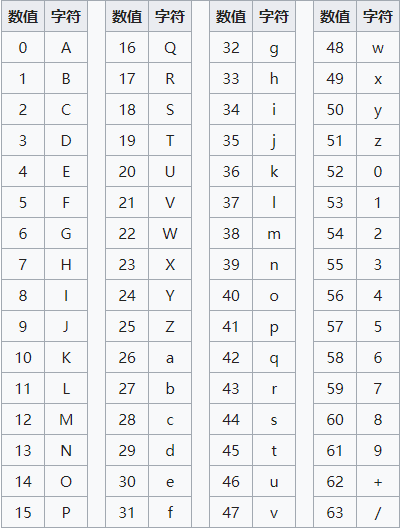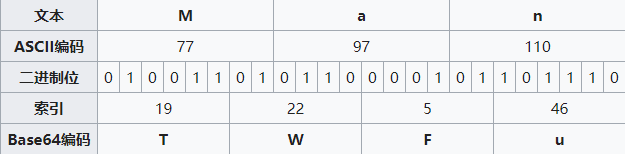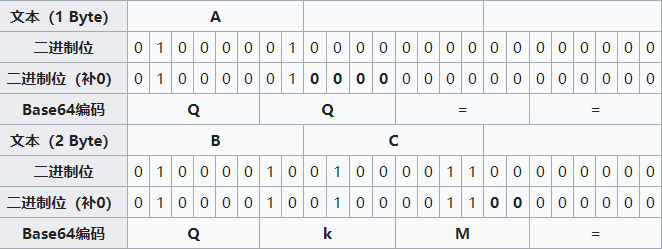# Base64原理以及隐写术

Posted by kevin on November 20, 2019

## Base64是什么## Base64转换## Base64 隐写1. = 去掉，剩下来的字符对着 Base64 编码表转换成相应的二进制，也就是

QkM= -> QkM ->  010000100100001100

2. 从左到右，按 8 个 bit 为一组，扔掉多余的位，将剩余的转换成 ASCII 码，也就是

01000010 01000011 00 -> 01000010 01000011 -> 66 67 -> BC


## Python Script

### 编码(有点小问题，留个坑)

import base64

flag = 'flag{base_64_is_funny}'
bin_str = ''.join([bin(ord(c)).replace('0b', '').zfill(8) for c in flag])
base64chars = 'ABCDEFGHIJKLMNOPQRSTUVWXYZabcdefghijklmnopqrstuvwxyz0123456789+/'

with open('raw.txt', 'r') as fin, open('encode.txt', 'w+') as fout:
row_str = str(base64.b64encode((line.replace('\n', '')).encode('utf-8')), 'utf-8')
equalnum = row_str.count('=')

if equalnum and len(bin_str):
offset = int('0b' + bin_str[: equalnum * 2], 2)
last_char = row_str[len(row_str) - equalnum - 1]    # the last character
row_str = row_str.replace(last_char, base64chars[base64chars.index(last_char) + offset])    # change the last character
bin_str = bin_str[equalnum * 2:]

fout.write(row_str + '\n')


### 解码

import base64

#coding=utf-8

base64chars = 'ABCDEFGHIJKLMNOPQRSTUVWXYZabcdefghijklmnopqrstuvwxyz0123456789+/'

#文件路径
with open('encode.txt', 'rb') as f:
flag = ''
bin_str = ''
stegb64 = str(line, 'utf-8').strip('\n')
rowb64 = str(base64.b64encode(base64.b64decode(stegb64)), 'utf-8').strip('\n')
offset = abs(base64chars.index(stegb64.replace('=', '')[-1]) - base64chars.index(rowb64.replace('=', '')[-1]))
equalnum = stegb64.count('=')  # no equalnum no offset
if equalnum:
bin_str += bin(offset)[2:].zfill(equalnum * 2)
res = [chr(int(bin_str[i:i + 8], 2)) for i in range(0, len(bin_str), 8)]
print(res)


## reference

https://zh.wikipedia.org/wiki/Base64

https://www.tr0y.wang/2017/06/14/Base64steg/